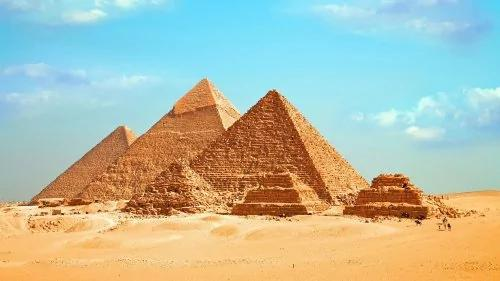﻿ 金字塔神奇數字「142857」蘊藏宇宙秘密？|☀阿波羅新聞網

# 金字塔神奇數字「142857」蘊藏宇宙秘密？「142857」這一組阿拉伯數字，看起來似乎沒什麼特別的地方，但往下看你就會發現它的神奇，因為它是一個「走馬燈數」（一個n位數乘以1至n的總數是原數的循環排列）。

142857×1=142857

142857×2=285714

142857×3=428571

142857×4=571428

142857×5=714285

142857×6=857142

142+857=999

14+28+57=99

20408+122449=142857

142857×1=142857（原數字）

142857×2=285714（輪值）

142857×3=428571（輪值）

142857×4=571428（輪值）

142857×5=714285（輪值）

142857×6=857142（輪值）

142857×7=999999（放假由9代班）

142857×8=1142856（7分身，變成頭尾的1和6，數列內少了7）

142857×9=1285713（4分身，變成頭尾的1和3，數列內少了4）

142857×10=1428570（1分身）

142857×11=1571427（8分身）

142857×12=1714284（5分身）

142857×13=1857141（2分身）

142857×14=1999998（9分身）

1+4+2+8+5+7=27=2+7=9

1+9+9+9+9+9+8=54=5+4=9